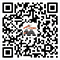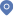首页 - 科研队伍• 研究方向：

可积系统与数值算法
• 代表作：

``` X. B. Hu, A powerful approach to generate new integrable systems. J. Phys. A 27(1994)(7), 2497–2514

 X.B. Hu, H.Y. Wang, Construction of dKP and BKP equations with self-consistent sources. Inverse Problems 22(2006)(5), 1903–1920.

 Y. He, X.B. Hu, J.Q. Sun, E.J. Weniger, Convergence acceleration algorithm via an equation related to the lattice Boussinesq equation. SIAM J. Sci. Comput. 33(2011)(3), 1234–1245.

 X.K. Chang, X.M. Chen, X.B. Hu, A generalized nonisospectral Camassa-Holm equation and its multipeakon solutions. Adv. Math. 263(2014), 154–177

 B. Wang, X.K. Chang, X.B. Hu, S.H. Li, Discrete invariant curve flows, orthogonal polynomials, and moving frame. IMRN 2021(2021)(14), 11050–11092```
• 联系方式：

办公室：中科院数学院南楼730房间 电　话：0086-10-82541928 邮　箱：hxb@lsec.cc.ac.cn